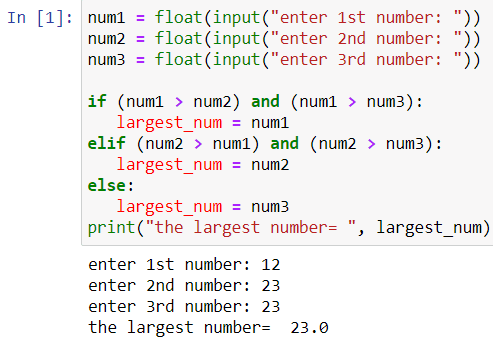# Python 3 Program to Find Largest Among Three Numbers

In this program, you will find the simple Python 3 program to find the largest among the three numbers. To understand this program, you must have basic knowledge of Python programming concepts such as variables, operators, and conditional statements. Here are some recommended Python books for beginners that you can use in your Python journey.

Recent Python TutorialHow To Send Email Using Python With File Attachments.

## Python 3 Program To Find The Largest Among Three Numbers

Take three numbers of input from the user using the input function. After that, you can use if – elif statements to compare three numbers and print the largest among the three numbers.

## Source Code

```num1 = float(input("enter 1st number: "))
num2 = float(input("enter 2nd number: "))
num3 = float(input("enter 3rd number: "))

if (num1 > num2) and (num1 > num3):
largest_num = num1
elif (num2 > num1) and (num2 > num3):
largest_num = num2
else:
largest_num = num3
print("the largest number= ", largest_num)```

## OutputScroll to Top